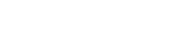# math.atanh

`FLOATmath.atanhFLOATx`

Available inall subroutines.

Computes the inverse hyperbolic tangent of its argument `x`.

## Parameters

`x` - Floating point value representing a hyperbolic angle.

## Return Value

Upon successful completion, this function returns the inverse hyperbolic tangent of `x`.

If `x` is `math.NAN`, a NaN will be returned.

If `x` is ±0, `x` will be returned.

If `x` is `math.POS_INFINITY` or `math.NEG_INFINITY`, a domain error occurs and a NaN will be returned.

If `x` is subnormal, a range error occurs and `x` will be returned.

For finite |`x`|>1, a domain error occurs and a NaN will be returned.

If `x` is ±1, a pole error occurs, and `math.atanh()` will return the value of the macro `math.POS_HUGE_VAL` or `math.NEG_HUGE_VAL` with the same sign as the result of the function.

## Errors

• If the `x` argument is finite and not in the range -1 to 1 inclusive, or if it is `math.POS_INFINITY` or `math.NEG_INFINITY`, then `fastly.error` will be set to `EDOM`.
• If the `x` argument is subnormal, or ±1, then `fastly.error` will be set to `ERANGE`.

## Example

``````declare local var.fo FLOAT;
set var.fo = math.atanh(-1); // Returns `math.NEG_INFINITY`
if (fastly.error) {  set resp.http.atanh-error = fastly.error; // Returns "ERANGE"}``````

BETA